# PACK

This operator, as well as the following one, unpack, maps (packs) sets of tuples in R, whose (n1) components for Ck are the same, into a single tuple.

Let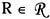be a relation included in the set of relations in the database, with |Atr (R) | = n, A Atr (R) and CA = Atr(R) {A}. For each (n-1)-tuple g CA (R), we define: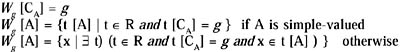then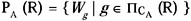For example, consider the relation R1 in Figure 22(a). The relations R3 and R4, where R3 = P1 (1,2,3 (R1)) and P4(R3), are shown in Figures 22(b) and (c).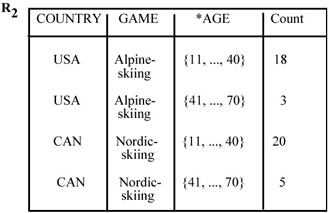Figure 22a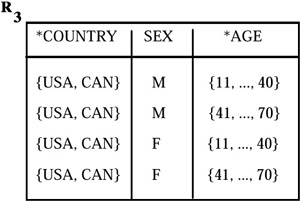Figure 22b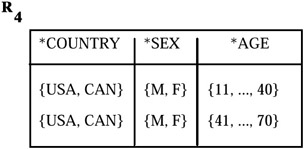Figure 22cMultidimensional Databases: Problems and Solutions
ISBN: 1591400538
EAN: 2147483647
Year: 2003
Pages: 150